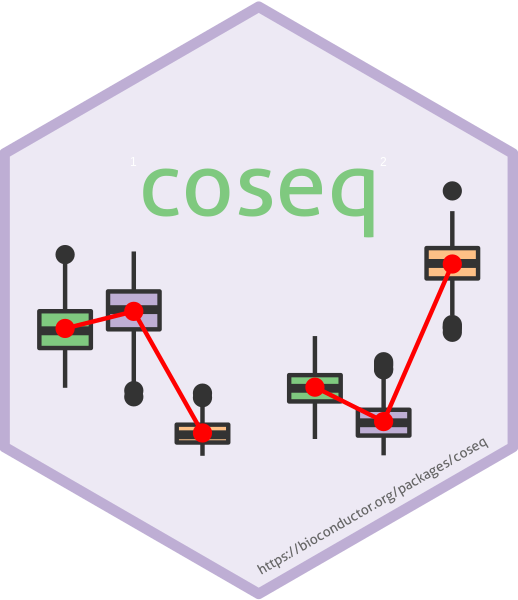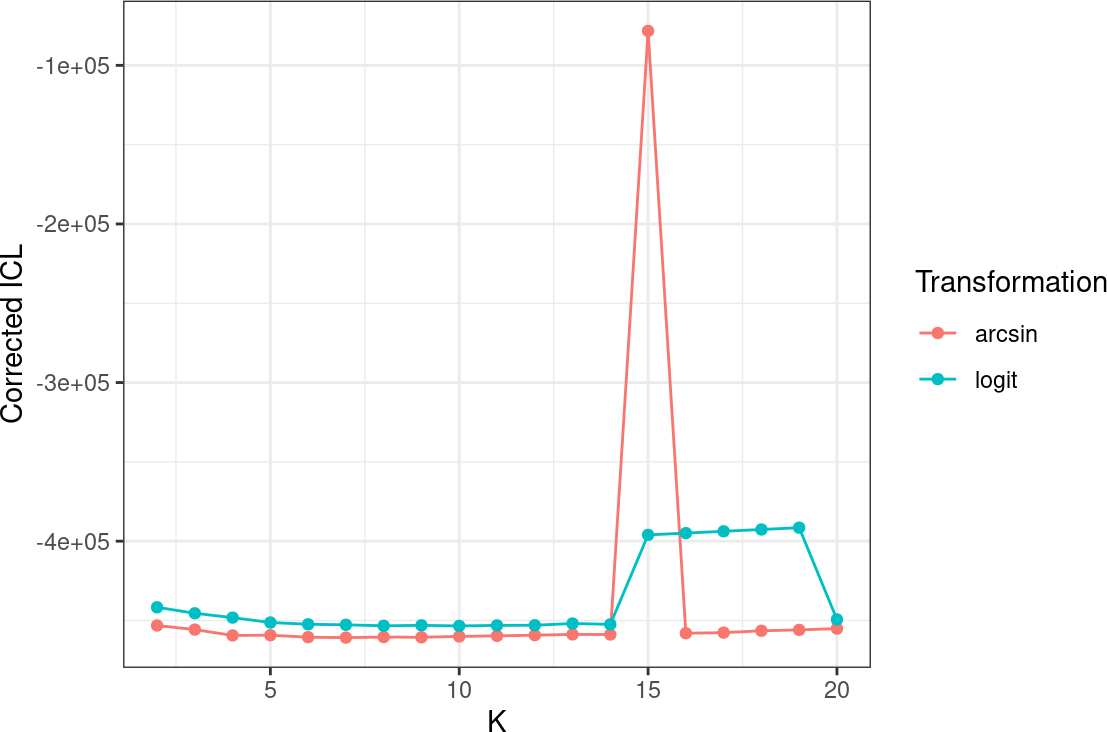coseq is a package to perform clustering analysis of sequencing data (e.g., co-expression analysis of RNA-seq data), using transformed profiles rather than the raw counts directly. The package implements two distinct strategies in conjunction with transformations: 1) MacQueen’s K-means algorithm and 2) Gaussian mixture models. For both strategies model selection is provided using the slope heuristics approach and integrated completed likelihood (ICL) criterion, respectively. Note that for backwards compatibility, coseq also provides a wrapper for the Poisson mixture model originally proposed in Rau et al. (2015) and implemented in the HTSCluster package.

The methods implemented in this package are described in detail in the following three publications.

• Godichon-Baggioni, A., Maugis-Rabusseau, C. and Rau, A. (2018) Clustering transformed compositional data using K-means, with applications in gene expression and bicycle sharing system data. Journal of Applied Statistics, doi:10.1080/02664763.2018.1454894. [Paper that introduced the use of the K-means algorithm for RNA-seq profiles after transformation via the centered log ratio (CLR) or log centered log ratio (logCLR) transformation.]

• Rau, A. and Maugis-Rabusseau, C. (2018) Transformation and model choice for co-expression analayis of RNA-seq data. Briefings in Bioinformatics, 19(3)-425-436. [Paper that introduced the idea of clustering profiles for RNA-seq co-expression, and suggested using Gaussian mixture models in conjunction with either the arcsine or logit transformation.]

• Rau, A., Maugis-Rabusseau, C., Martin-Magniette, M.-L., Celeux, G. (2015) Co-expression analysis of high-throughput transcriptome sequencing data with Poisson mixture models. Bioinformatics, 31(9): 1420-1427. link [Paper that introduced the use of Poisson mixture models for RNA-seq counts.]

Below, we provide a quick-start guide using a subset of RNA-seq data to illustrate the functionalities of the coseq package. In this document, we focus on the methods described in Rau and Maugis-Rabusseau (2018) and Godichon-Baggioni et al. (2018). For more information about the method described in Rau et al. (2015), see the HTSCluster vignette.

# 1 Quick start (tl;dr)

A standard coseq analysis takes the following form, where counts represents a matrix or data.frame of gene-level counts arising from an RNA-seq experiment (of dimension n x d for n genes and d samples). Results, exported in the form of a coseqResults S4 object, can easily be examined using standard summary and plot functions (see below and the User’s Guide for examples of plots that may be obtained):

run <- coseq(counts, K=2:25)
summary(run)
plot(run)

The cluster labels for the selected model obtained from the coseq clustering may easily be obtained using the clusters function:

clusters(run)

Similarly, the conditional probabilities of cluster membership for each gene in the selected coseq clustering model may be obtained using the assay accessor function:

assay(run)

Note that unless otherwise specified by the user, coseq uses the following default parameters:

• K-means algorithm
• Log centered log transformation (logclr) on profiles
• Library size normalization via the Trimmed Mean of M-values (TMM) approach
• Model selection (choice of the number of clusters) via the slope heuristics approach
• No parallelization.

Note that rerunning coseq may lead to different results (both for the number of chosen clusters as well as the cluster assignments) due to differences in initialization. For reproducible results, make sure to use the seed argument to fix the seed of the random number generator:

run <- coseq(counts, K=2:25, seed=12345)

# 2 Co-expression analysis with coseq

For the remainder of this vignette, we make use of the mouse neocortex RNA-seq data from Fietz et al. (2012) (available at https://perso.math.univ-toulouse.fr/maugis/mixstatseq/packages and as an ExpressionSet object called fietz in coseq). The aim in this study was to investigate the expansion of the neocortex in five embryonic (day 14.5) mice by analyzing the transcriptome of three regions: the ventricular zone (VZ), subventricular zone (SVZ) and cortical place (CP).

We begin by first loading the necessary R packages as well as the data.

library(coseq)
library(Biobase)
library(corrplot)

data("fietz")
counts <- exprs(fietz)
conds <- pData(fietz)\$tissue

## Equivalent to the following:
## conds <- c(rep("CP",5),rep("SVZ",5),rep("VZ",5))

The coseq package fits either a Gaussian mixture model (Rau and Maugis-Rabusseau, 2018) or uses the K-means algorithm (Godichon-Baggioni et al., 2018) to cluster transformed normalized expression profiles of RNA-seq data. Normalized expression profiles correspond to the proportion of normalized reads observed for gene i with respect to the total observed for gene i across all samples: $p_{ij} = \frac{y_{ij}/s_{j} +1}{\sum_{j'} y_{ij'}/s_{j'} +1},$ where $$s_j$$ are normalization scaling factors (e.g., after applying TMM normalization to library sizes) and $$y_{ij}$$ represents the raw count for gene $$i$$ in sample $$j$$.

## 2.1 Transformations for normalized profiles with the Gaussian mixture model

Since the coordinates of $$\mathbf{p}_i$$ are linearly dependent (causing estimation problems for a Gaussian mixture distribution), weconsider either the arcsine or logit transformation of the normalized profiles $$p_{ij}$$: $g_{\text{arcsin}}(p_{ij}) = \text{arcsin}\left( \sqrt{ p_{ij} } \right) \in [0, \pi/2], \text{ and}$ $g_{\text{logit}}(p_{ij}) = \text{log}_2 \left( \frac{p_{ij}}{1-p_{ij}} \right) \in (-\infty, \infty).$

Then the distribution of the transformed normalized expression profiles is modeled by a general multidimensional Gaussian mixture

$f(.|\theta_K) = \sum_{k=1}^K \pi_k \phi(.|\mu_k,\Sigma_k)$

where $$\theta_K=(\pi_1,\ldots,\pi_{K-1},\mu_1,\ldots,\mu_K,\Sigma_1,\ldots,\Sigma_K)$$, $$\pi=(\pi_1,\ldots,\pi_K)$$ are the mixing proportions and $$\phi(.|\mu_k,\Sigma_k)$$ is the $$q$$-dimensional Gaussian density function with mean $$\mu_k$$ and covariance matrix $$\Sigma_k$$. To estimate mixture parameters $$\theta_K$$ by computing the maximum likelihood estimate (MLE), an Expectation-Maximization (EM) algorithm is used via the Rmixmod package. Finally, let $$\hat t_{ik}$$ be the conditional probability that observation $$i$$ arises from the $$k$$th component of the mixture $$f(.|\hat \theta_{\hat K})$$. Each observation $$i$$ is assigned to the component maximizing the conditional probability $$\hat t_{ik}$$ i.e., using the so-called maximum a posteriori (MAP) rule.

## 2.2 Transformations for normalized profiles with K-means

For the K-means algorithm, we consider three separate transformations of the profiles $$p_{i}$$ that are well adapted to compositional data (see Godichon-Baggioni et al., 2017 for more details):

• Identity (i.e., no transformation)
• Centered log ratio (CLR) transformation
• Log centered log ratio (logCLR) transformation

Then, the aim is to minimize the sum of squared errors (SSE), defined for each set of clustering $$\mathcal{C}^{(K)}=\left( C_{1},...,C_{k}\right)$$ by $\begin{equation*} \text{SSE} \left( \mathcal{C}^{(K)}\right) := \sum_{k=1}^{K}\sum_{i \in C_{k}} \left\| h \left( y_{i}\right) - \mu_{k,h} \right\|_{2}^{2} , \end{equation*}$ with $$i \in C_{k}$$ if $$\left\| h\left( y_{i}\right) - \mu_{k,h} \right\|_{2} = \min_{k'=1,\ldots,K} \left\| y_{i}- \mu_{k',h} \right\|_{2}$$, and $\mu_{k,h}= \frac{1}{|C_{k} |}\sum_{i \in C_{k}}h \left( y_{i} \right),$ and $$h$$ is the chosen transformation. In order to minimize the SSE, we use the well-known MacQueen’s K-means algorithm.

## 2.3 Model selection

Because the number of clusters $$K$$ is not known a priori, we fit a collection of models (here $$K$$ = 2, …, 25) and use either the Integrated Completed Likelihood (ICL) criterion (in the case of the Gaussian mixture model) or the slope heuristics (in the case of the K-means algorithm) to select the best model in terms of fit, complexity, and cluster separation.

## 2.4 Other options

If desired, we can set a filtering cutoff on the mean normalized counts via the meanFilterCutoff argument to remove very weakly expressed genes from the co-expression analysis; in the interest of faster computation for this vignette, in this example we filter all genes with mean normalized expression less than 200 (for the Gaussian mixture model) or less than 50 (for the K-means algorithm).

Note that if desired, parallel execution using BiocParallel can be specified via the parallel argument:

run <- coseq(..., parallel=TRUE)

# 3 Running coseq

The collection of co-expression models for the logCLR-transformed profiles using the K-means algorithm may be obtained as follows (note that we artificially set the number of maximum allowed iterations and number of random starts to be low for faster computational time in this vignette):

runLogCLR <- coseq(counts, K=2:25, transformation="logclr",norm="TMM",
meanFilterCutoff=50, model="kmeans",
nstart=1, iter.max=10)
## ****************************************
## coseq analysis: kmeans approach & logclr transformation
## K = 2 to 25
## Use seed argument in coseq for reproducible results.
## ****************************************
## Running K = 2 ...
## Running K = 3 ...
## Running K = 4 ...
## Running K = 5 ...
## Running K = 6 ...
## Running K = 7 ...
## Running K = 8 ...
## Running K = 9 ...
## Running K = 10 ...
## Running K = 11 ...
## Running K = 12 ...
## Running K = 13 ...
## Running K = 14 ...
## Running K = 15 ...
## Running K = 16 ...
## Running K = 17 ...
## Running K = 18 ...
## Running K = 19 ...
## Running K = 20 ...
## Running K = 21 ...
## Running K = 22 ...
## Running K = 23 ...
## Running K = 24 ...
## Running K = 25 ...

The collection of Gaussian mixture models for the arcsine-transformed and logit-transformed profiles may be obtained as follows (as before, we set the number of iterations to be quite low for computational speed in this vignette):

runArcsin <- coseq(counts, K=2:20, model="Normal", transformation="arcsin",
meanFilterCutoff=200, iter=10)
## ****************************************
## coseq analysis: Normal approach & arcsin transformation
## K = 2 to 20
## Use seed argument in coseq for reproducible results.
## ****************************************
## Running K = 2 ...
## Running K = 3 ...
## Running K = 4 ...
## Running K = 5 ...
## Running K = 6 ...
## Running K = 7 ...
## Running K = 8 ...
## Running K = 9 ...
## Running K = 10 ...
## Running K = 11 ...
## Running K = 12 ...
## Running K = 13 ...
## Running K = 14 ...
## Running K = 15 ...
## Running K = 16 ...
## Running K = 17 ...
## Running K = 18 ...
## Running K = 19 ...
## Running K = 20 ...
runLogit <- coseq(counts, K=2:20, model="Normal", transformation="logit",
meanFilterCutoff=200, verbose=FALSE, iter=10)
## ****************************************
## coseq analysis: Normal approach & logit transformation
## K = 2 to 20
## Use seed argument in coseq for reproducible results.
## ****************************************

In all cases, the resulting output of a call to coseq is an S4 object of class coseqResults.

class(runArcsin)
##  "coseqResults"
## attr(,"package")
##  "coseq"
runArcsin
## An object of class coseqResults
##  4230 features by 15 samples.
##  Models fit: K = 2 ... 20
##  Chosen clustering model: K = 7

To choose the most appropriate transformation to use (arcsine versus logit) in a Gaussian mixture model, we may use the corrected ICL, where the minimum value corresponds to the selected model. Note that this feature is not available for the K-means algorithm.

compareICL(list(runArcsin, runLogit))This indicates that the preferred transformation is the arcsine, and the selected model has K = 7 clusters. We can additionally explore how similar the models with K = 8 to 12 are using the adjusted Rand index (ARI) via the compareARI function. Values close to 1 indicate perfect agreement, while values close to 0 indicate near random partitions.

compareARI(runArcsin, K=8:12)